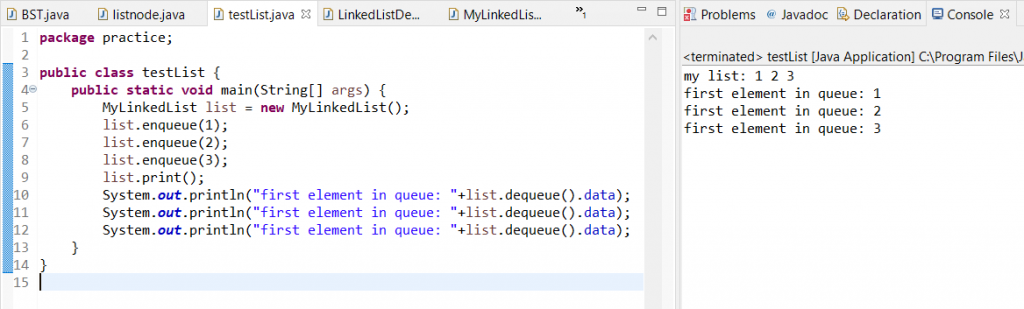A Java LinkedList is a linear data structure. As the name suggests, it is a List of data linked with each other. A linked list is a common data structure and has its implementations in almost every language. The idea is the same everywhere with a slight variation of flavor.

## Linked List in Java

In Linked List, we are going to defines each nodes with data and a self-referential structure to contain the next node. The first node will be named as head and it will be the access point of the entire list! Therefore, if we need to access any element in the middle or end of the list we shall iterate through the list until we find the required node. In Java, we can use the ready-made class named LinkedList from util package. For instance,

``````import java.util.*;
public class LinkedListDemo {
public static void main(String[] args) {
List mylist = new LinkedList();
System.out.println(mylist);
}
}
``````

OUTPUTBut here we shall learn how to create a LinkedList of our own!First, we must define a Node. each combination of data and next must be defined in a class called “node”.

``````public class listnode {
int data;
listnode next;
}``````

This class will have a global variable that will serve as the head node for our List.

``````public class MyLinkedList {

• insertion
• print
• deletion

### insertion( ) method Java LinkedList

``````void insertion(int data) {
listnode newnode = new listnode();
newnode.data = data;

if(head == null) {
}
else {
listnode temp = head;
while(temp.next != null) {
temp = temp.next;
}
temp.next = newnode;
}
}``````

On line 4, we check if the head value is null? If true, it indicates that it is the first insertion in the list. Therefore, we simply, assign the ‘newnode’ as head for the List.

However, from the 2nd insertion and so on, we iterate to the end of the list by checking for null in the next of current node using ‘if (temp.next == null)’. When reached the end, we link the new node at the tail of the list.

### print( ) method in Java LinkedList

`````` void print() {
listnode temp = head;
System.out.print("my list: ");
while(temp != null){
System.out.print(temp.data+" ");
temp = temp.next;
}
}``````

Finally, this function is used to print LinkedList in Java. We iterate the end of the list using a similar logic and print the data every time.

### deletion( ) method in Java LinkedList

In the deletion function, we get 3 cases:

2. in-between
3. at the end
`````` void delete(int data) {
listnode temp = head;
if(temp.data == data) {
return;
}
while(temp.next != null) {
if(temp.next.data == data) {
temp.next = temp.next.next;
}
if(temp.next == null)
return;
temp = temp.next;
}

}``````

For instance, in line 3 we check for the first case, delete the first element. Then in the while block, we search if temp next data matches with the data to be deleted. If true we assign the value of temp.next.next to temp next.The second if block checking if temp next is null or not, is very important. It is used for the 3rd case, or else a `NullPointerException` will be thrown.

### ListNode

``````public class listnode {
int data;
listnode next;
}
``````

``````public class MyLinkedList {
void insertion(int data) {
listnode newnode = new listnode();
newnode.data = data;

if(head == null) {
}
else {
listnode temp = head;
while(temp.next != null) {
temp = temp.next;
}
temp.next = newnode;
}
}

void delete(int data) {
listnode temp = head;
if(temp.data == data) {
return;
}
while(temp.next != null) {
if(temp.next.data == data) {
temp.next = temp.next.next;
}
if(temp.next == null)
return;
temp = temp.next;
}

}

void print() {
listnode temp = head;
System.out.print("my list: ");
while(temp != null){
System.out.print(temp.data+" ");
temp = temp.next;
}
System.out.println();
}
}
``````

#### Driver class

``````public class testList {
public static void main(String[] args) {
list.insertion(1);
list.insertion(2);
list.insertion(3);
list.print();
list.delete(1);//deletes the head node
list.print();
}
}``````

OUTPUTdelete the first element in LinkedList java

A Queue is a linear Data structure that follows the FIFO mechanism. First in First out. In other words, it behaves just like a normal queue we see in our day-to-day lives.

To insert a value in the queue, we use the `enqueue` function. However, it is exactly the same as the insertion function we created for our Linked List.

### enqueue( )

We shall insert the queue elements one after the other.

`````` void enqueue(int data) {
listnode newnode = new listnode();
newnode.data = data;

if(head == null) {
}
else {
listnode temp = head;
while(temp.next != null) {
temp = temp.next;
}
temp.next = newnode;
}
}``````

### dequeue( )

In this function, we will return the head node and then delete it using the previous delete function.

``````listnode dequeue() {
listnode temp = head;
return temp;
}``````

#### Driver Code

``````public class testList {
public static void main(String[] args) {
list.enqueue(1);
list.enqueue(2);
list.enqueue(3);
list.print();
System.out.println("first element in queue: "+list.dequeue().data);
System.out.println("first element in queue: "+list.dequeue().data);
System.out.println("first element in queue: "+list.dequeue().data);
}
}``````

OUTPUTenqueue and dequeue in java LinkedList

We hope this tutorial helped you to achieve the same. Keep learning keep sharing. Follow us on Facebook and Instagram. Also, if you need any help with Java coding help, feel free to contact us.